# Common Core: 3rd Grade Math : Solve Real World and Mathematical Problems Involving Area: CCSS.Math.Content.3.MD.C.7b

## Example Questions

← Previous 1 3 4 5 6

### Example Question #1 : Solve Real World And Mathematical Problems Involving Area: Ccss.Math.Content.3.Md.C.7b

What is the area of the rectangle?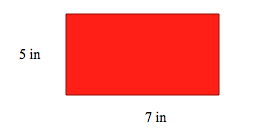Explanation:

The formula to find area is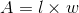. We are given the length and the width from the problem, so we can plug those values into our equation and solve.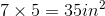*Area is the number of square units inside a shape, which is why area is always written with square units.

### Example Question #2 : Solve Real World And Mathematical Problems Involving Area: Ccss.Math.Content.3.Md.C.7b

What is the area of the rectangle?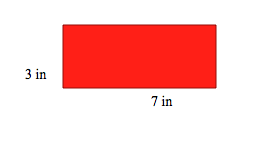Explanation:

The formula to find area is. We are given the length and the width from the problem, so we can plug those values into our equation and solve.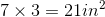*Area is the number of square units inside a shape, which is why area is always written with square units.

### Example Question #3 : Solve Real World And Mathematical Problems Involving Area: Ccss.Math.Content.3.Md.C.7b

What is the area of the rectangle?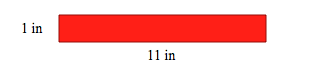Explanation:

The formula to find area is. We are given the length and the width from the problem, so we can plug those values into our equation and solve.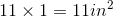*Area is the number of square units inside a shape, which is why area is always written with square units.

### Example Question #4 : Solve Real World And Mathematical Problems Involving Area: Ccss.Math.Content.3.Md.C.7b

What is the area of the rectangle?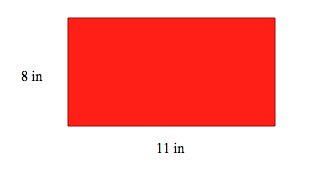Explanation:

The formula to find area is. We are given the length and the width from the problem, so we can plug those values into our equation and solve.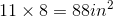*Area is the number of square units inside a shape, which is why area is always written with square units.

### Example Question #1 : Solve Real World And Mathematical Problems Involving Area: Ccss.Math.Content.3.Md.C.7b

What is the area of the rectangle?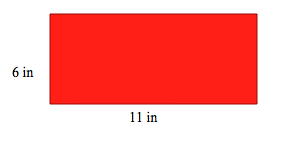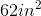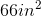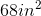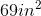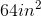Explanation:

The formula to find area is. We are given the length and the width from the problem, so we can plug those values into our equation and solve.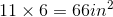*Area is the number of square units inside a shape, which is why area is always written with square units.

### Example Question #6 : Solve Real World And Mathematical Problems Involving Area: Ccss.Math.Content.3.Md.C.7b

What is the area of the rectangle?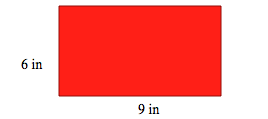Explanation:

The formula to find area is. We are given the length and the width from the problem, so we can plug those values into our equation and solve.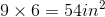*Area is the number of square units inside a shape, which is why area is always written with square units.

### Example Question #7 : Solve Real World And Mathematical Problems Involving Area: Ccss.Math.Content.3.Md.C.7b

What is the area of the rectangle?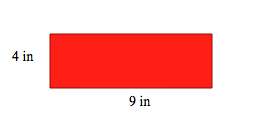Explanation:

The formula to find area is. We are given the length and the width from the problem, so we can plug those values into our equation and solve.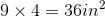*Area is the number of square units inside a shape, which is why area is always written with square units.

### Example Question #8 : Solve Real World And Mathematical Problems Involving Area: Ccss.Math.Content.3.Md.C.7b

What is the area of the rectangle?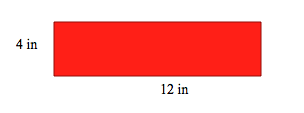Explanation:

The formula to find area is. We are given the length and the width from the problem, so we can plug those values into our equation and solve.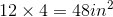*Area is the number of square units inside a shape, which is why area is always written with square units.

### Example Question #9 : Solve Real World And Mathematical Problems Involving Area: Ccss.Math.Content.3.Md.C.7b

What is the area of the rectangle?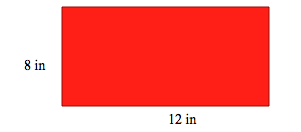Explanation:

The formula to find area is. We are given the length and the width from the problem, so we can plug those values into our equation and solve.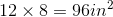*Area is the number of square units inside a shape, which is why area is always written with square units.

### Example Question #2 : Solve Real World And Mathematical Problems Involving Area: Ccss.Math.Content.3.Md.C.7b

What is the area of the rectangle?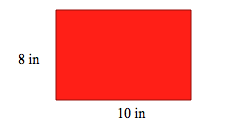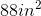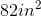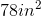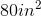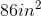Explanation:

The formula to find area is. We are given the length and the width from the problem, so we can plug those values into our equation and solve.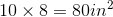*Area is the number of square units inside a shape, which is why area is always written with square units.

← Previous 1 3 4 5 6

### All Common Core: 3rd Grade Math Resources